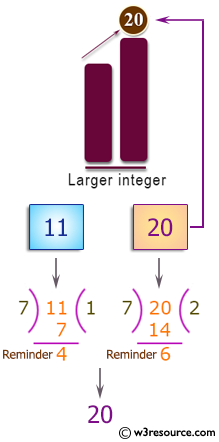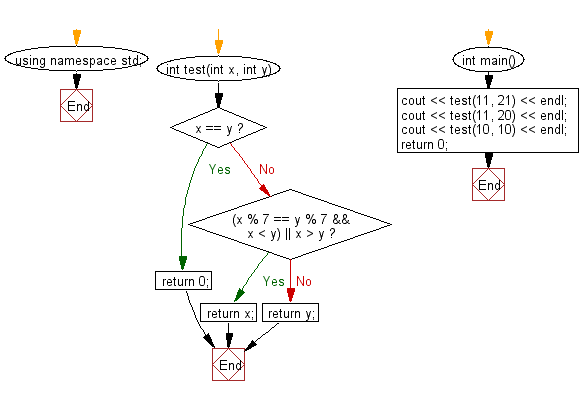﻿ C++ Exercises: Find the larger from two given integers - w3resource# C++ Exercises: Find the larger from two given integers

## C++ Basic Algorithm: Exercise-49 with Solution

Write a C++ program to find the larger from two given integers. However if the two integers have the same remainder when divided by 7, then the return the smaller integer. If the two integers are the same, return 0.

Sample Solution:

C++ Code :

``````#include <iostream>
using namespace std;

int test(int x, int y)
{
if (x == y)
{
return 0;
}
else if ((x % 7 == y % 7 && x < y) || x > y)
{
return x;
}
else
{
return y;
}
}

int main()
{
cout << test(11, 21) << endl;
cout << test(11, 20) << endl;
cout << test(10, 10) << endl;
return 0;
}
``````

Sample Output:

```21
20
0
```

Pictorial Presentation:Flowchart:C++ Code Editor: